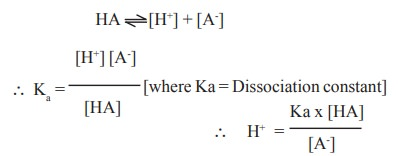Home | | Biochemistry | Acids and bases

# Acids and bases

According to the modern concept, an acid is defined as that species which can donate H+ ions (protons) in solution and a base is that species which can accept H+ ions.

Acids and bases

According to the modern concept, an acid is defined as that species which can donate H+ ions (protons) in solution and a base is that species which can accept H+ ions. Since such transfer of protons is reversible any acid which gives up its proton becomes a base, while any base which accepts a proton becomes an acid. This theory was postulated by Bronsted and Lowry in 1923. The following general equation can be written asAn acid and a base related in this manner are called conjugates

For (eg) in this reactionAn acid which dissociates strongly and readily gives H+ ions is known as a strong acid. The capacity of an acid to release its protons is known as acidity.

A base which has more affinity to combine with H+ ions is known as a strong base.

This property of a base is known as alkalinity (eg) HCO3-, HSO4-, H2PO4- etc.

The acidity of a species is denoted by its pH value : larger the acidity of a species, lower will be its pH value.

pH - It is defined as the negative logarithm of hydrogen ion concentration (or) it is defined as the logarithm of reciprocal of the hydrogen ion concentration.

i.e. pH = - log [H+] (or) pH = log 1/[H+]

Acidic - 1 - 6.9 Neutral 7.0 Alkaline 7.1 - 14.

## Determination of pH -Henderson - Hasselbalch equation Derivation

This concerns the dissociation of weak acid in equilibrium.

Let us consider HA a weak acid that ionises as follows:log [H+] = log Ka + log[HA]-log[A-] (Taking log on both sides).

-log [H+] = -log Ka -log [HA] + [A-] [Chaning sign on both sides].

pH = pKa + log [A-] / [HA] since -log H+ = pH and - log Ka = pKa

The above equation is known as Henderson - Hasselbalch equation. and can be used for the determination of pH of blood.

## Determination of pH of buffers

The pH of buffers can be determined by Henderson - Hasselbalch equation.

pH = pKa + log [salt] / [Acid]In case of blood, the ratio between [BHCO3] : [H2CO3] can be found out by applying the above equation to maintain average pH of blood 7.4. If the pKa value of H2CO3 is 6.1. then## pH in living organisms

The fluid in the cells and tissues of plants and animals is usually close to neutrality and the pH of extracellular fluids under normal conditions varies from 7.35 to 7.5.

## Regulations of acid-base balance (pH maintenance)

The following mechanisms control the regulation of acid-base balance in the human body.

1. Buffer system : Hemoglobin, phosphate and carbonate - bicarbonate buffers are involved in the maintenance of pH since they are capable of neutralising H+ or OH- ions formed during metabolic activities.

2. Respiratory regulation : Lungs  are  actually  the  most  effective  organs  for  pH

adjustment : One half of the H+ ions drained by the cells to the extra cellular fluids combine with HCO3 - to form H2CO3 which dissociates in to H2O and CO2. The CO2 thus formed is expirated through lungs. So accumulation of H+ ions is prevented.

3. Renal regulation

Kidney contribute for acid-base balance by excreting acids and ammonia. The excess of acids produced in the metabolic pathways are eliminated by kidneys in the form of urine. Ammonia is a base and formed in the tubular epithelial cells from glutamine. Glutamine is deaminated and then dehydrogenated to form two moles of ammonia. The ammonia so formed diffuses into the tubular urine and binds H+ ions to form NH4+ and excreted as such in urine. If not excreted, ammonia accumulation in blood leads to acid-base imbalance.

If acids and ammonia accumulate in the blood, it lead to acidosis and alkalosis respectively.

Study Material, Lecturing Notes, Assignment, Reference, Wiki description explanation, brief detail
Biochemistry: Living Cell : Acids and bases |